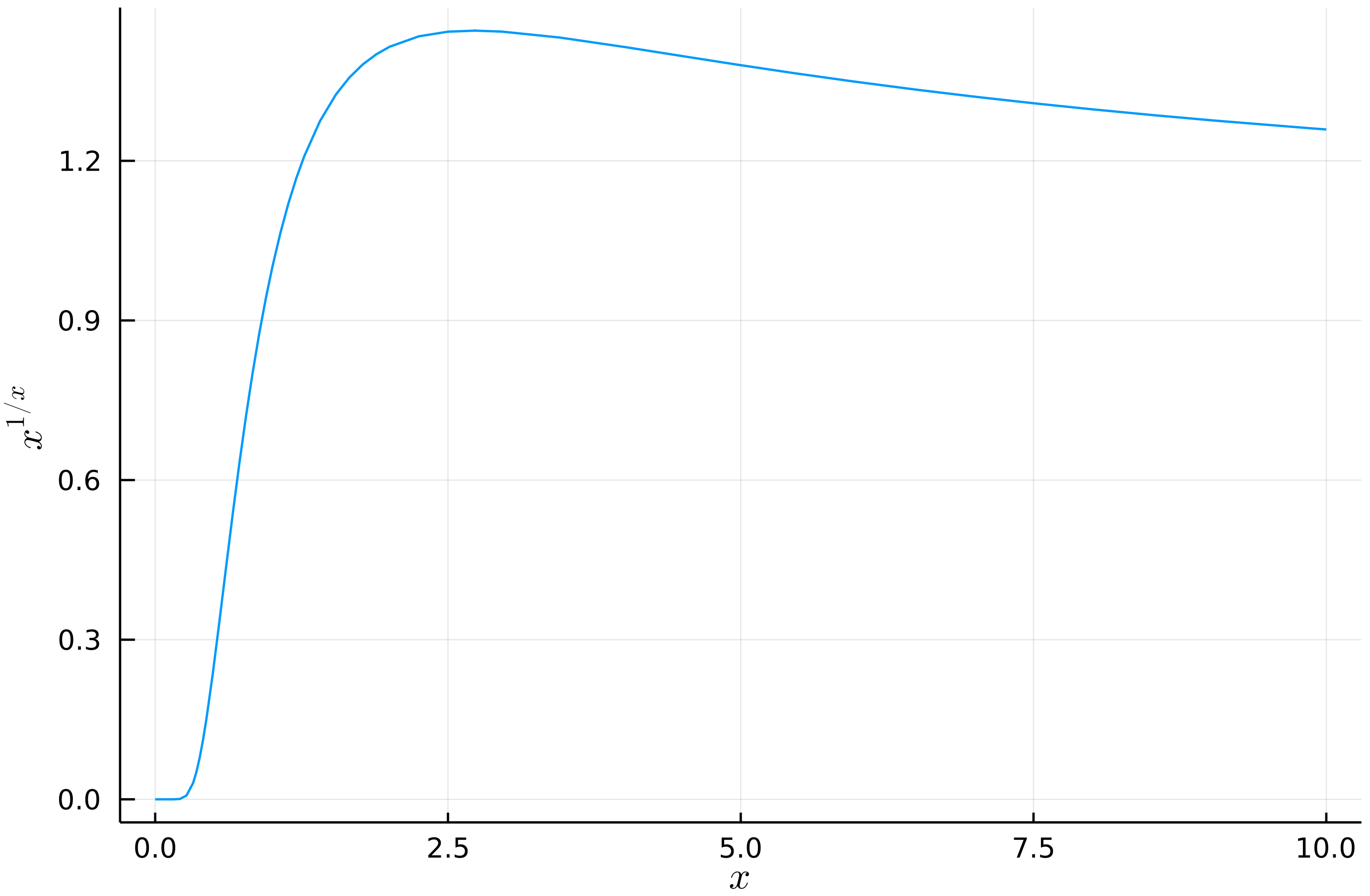# Introduction

After an amazing JuliaCon 2021 this week I thought to cover some lightweight topic in my post.

An interesting question I recently was asked is to find $$x\in\mathbf{R}^+\cup\{0\}$$ that maximizes $$x^{1/x}$$ (assuming that in $$0$$ the value of the function is established by the limit as $$x\to0^+$$).

As usual I will want to use Julia to get some insight into this problem before giving it a try.

This post was tested under Julia 1.6.1, Plots.jl 1.20.0, Optim.jl 1.4.1, and Symbolics.jl 2.0.

# Plotting

We start with plotting of the function we want to investigate:

julia> using Plots

julia> plot(x -> x^(1/x), 0, 10, legend=nothing,
xlab=raw"$x$", ylab=raw"$x^{1/x}$")It seems that the maximum is achieved around $$2.5$$, but we want to get more a detailed answer.

# Optimizing

Now we switch to optimization to find the value that maximizes our function numerically. Note that I optimize x -> -x^(1/x) (negated), as optim by default is looking for a minimum of a function.

julia> using Optim

julia> optimize(x -> -x^(1/x), 0, 10)
Results of Optimization Algorithm
* Algorithm: Brent's Method
* Search Interval: [0.000000, 10.000000]
* Minimizer: 2.718282e+00
* Minimum: -1.444668e+00
* Iterations: 13
* Convergence: max(|x - x_upper|, |x - x_lower|) <= 2*(1.5e-08*|x|+2.2e-16): true
* Objective Function Calls: 14


The optimal solution looks to be equal to $$e$$. Let us verify this using calculus.

# Solving

Now we check if the derivative of our function is equal to zero for $$e$$ as an argument.

julia> using Symbolics

julia> @variables x
1-element Vector{Num}:
x

julia> ex = Differential(x)(x^(1/x)) |> expand_derivatives |> simplify
x^(x^-1 - 2) - (log(x)*(x^(x^-1 - 2)))

julia> substitute(ex, x => MathConstants.e)
0.0


From the form of ex we also see that $$e$$ is the only zero of our derivative for positive values of $$x$$.

Now I am convinced enough about the result to try proving it using some elementary arguments.

# Pen and paper

Note that:

$x^{1/x} = e^{\log(x)/x}$

As exponential function is increasing it is enough to analyze $$\log(x)/x$$, which hopefully will be simpler. Substitute $$x = e^y$$, where $$x\to0^+$$ as $$y\to-\infty$$. With this substitution we get that we need to analyze:

$\frac{y}{e^y}.$

Now notice that as $$y\to-\infty$$ the fraction tends to $$-\infty$$, so our original expression $$x^{1/x}$$ tends to $$0^+$$ as $$x\to0^+$$. Similarly if $$y\to+\infty$$ the fraction tends to $$0^+$$, so $$x^{1/x}$$ tends to $$1$$ as $$x\to+\infty$$.

Having done this warm up task let us ask what $$y$$ maximizes $$y/e^y$$. From our earlier analyses we postulate that it is maximized for $$y=1$$, so we want to show that:

$\frac{y}{e^y} \leq \frac{1}{e}$

or, equivalently, substituting $$z=y-1$$:

$1+z \leq e^z.$

This is a well known consequence of Bernoulli’s inequality and the equality holds for $$z=0$$, which translates to $$x=e$$.

# Conclusions

I hope you enjoyed the post. Next week I will be back with DataFrames.jl related topics, so stay tuned.